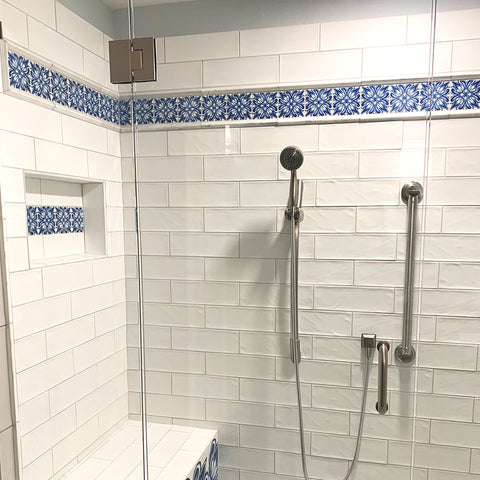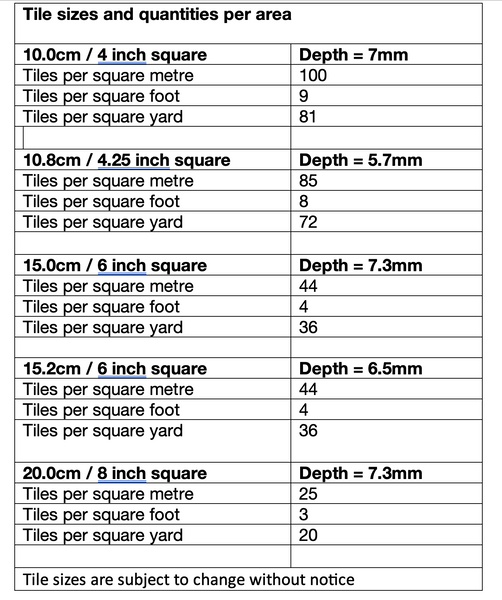# How many tiles do I need? Advice on calculating tile quantities.Tiles are expensive, so you don't want to order a lot more than you need.

But you also don't want to get to the end of a splashback and find you are three short!

With a tape measure and a bit of basic maths you can work out the area you plan to cover, and from that, how many tiles you need in your chosen size.

(This advice is taken from my ebook 'Using Pattern Tiles Effectively' which is available to download here.)

1: Working out quantities for a row

If you're doing a single row of tiles, you just need to divide the length of the row by the size of the tiles you've chosen.

So, if you've decided on a 6 inch tile, and you've got a 48 inch long space, you would need 8x 6 inch tiles (48 divided by 6).

If you're planning a double or triple height row, simply double or triple the quantity.2: Working out quantities for a panel or more complicated area.

For a larger area, you need to measure the length and width of the area.

If you work in Metric (centimetres and metres)

1. measure the height and width of the area to be tiled in centimetres

2. multiply the length by the height

3. divide by 10,000 - this will give you the area in square metres.

4. decide which tile size you'd like

5. Check how many tiles of your chosen size you need for one square metre (see table below)

6. Multiply the square metre figure, by this number of tiles = total number of tiles required.

7. So to cover 2 square metres with 15cm tiles is 2x44 = 88 tiles

If you work in Imperial (inches and feet)

1. measure the height and width of your area in inches.

2. multiply the length by the height.

3. divide by 144 - this will give you the area in square feet

4. decide which tile size you'd like (see table on next page)

5.Check how many tiles of your chosen size you need for one square foot (see table above)

6. Multiply the square feet, by this number of tiles = total number of tiles required

7. So to cover 4 square feet with 15cm tiles is 4x4 =16 tilesIf you have a complex area, the easiest way is to draw a diagram, and then divide the area up into rectangles.

You can then calculate the area of each rectangle (using the method above) and add them together to give your total area.

If you’re tiling a whole wall which has cabinets or other elements which won't be tiled, work out the entire area of the wall, and then measure the cabinets' area and subtract that from the whole wall (easier than measuring ‘around’ them!).

OK, so I've worked out how many I need. But do I need to order extras?

However, if your tiling project is relatively simple - for example a straightforward panel or row, without much in the way of cutting - you can probably get away with 5% or even less. (Just try not to drop any!).

If you know you're going to have to cut quite a few of the tiles, buying some extras is a very good idea. Cutting isn't difficult but tiles do shatter or break awkwardly, which means having some replacements on hand is very handy.

Some designs - for example Ocean Shoal large scale - need more spares than others. This is because the design is formed from four tiles, each with a different design. So if you break one, you may not have any spares of that particular pattern.

This is even more true for Fired Ink tiles, which take ages to make. You don't want to be waiting for a month for a single replacement tile!Fired Ink tiles are normally different to the above, and are available in a very wide range of sizes.

My standard sizes are:
Stated 10cm (actual 9.9cm) - 6.35mm deep
Stated 15cm (actual 14.9cm) - 6.7mm deep
Stated 20cm (actual 20cm) - 6.45mm
NB these sizes are subject to change without notice.